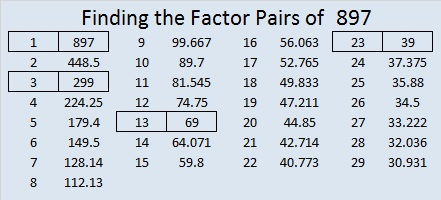# 897 and Level 1

(3 + 0)(3 + 10)(3 + 20) = 897

897 is the hypotenuse of a Pythagorean triple:

• 345-828-897, which is (5-12-13) times 69.Print the puzzles or type the solution on this excel file: 10-factors-897-904

• 897 is a composite number.
• Prime factorization: 897 = 3 × 13 × 23
• The exponents in the prime factorization are 1, 1, and 1. Adding one to each and multiplying we get (1 + 1)(1 + 1)(1 + 1) = 2 × 2 × 2 = 8. Therefore 897 has exactly 8 factors.
• Factors of 897: 1, 3, 13, 23, 39, 69, 299, 897
• Factor pairs: 897 = 1 × 897, 3 × 299, 13 × 69, or 23 × 39
• 897 has no square factors that allow its square root to be simplified. √897 ≈ 29.949958.